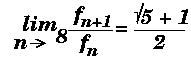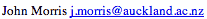Data Structures and Algorithms 9 Dynamic Algorithms

Sometimes, the divide and conquer approach seems appropriate but fails to produce an efficient algorithm.

We all know the algorithm for calculating Fibonacci numbers:

```int fib( int n ) {
if ( n < 2 ) return n;
else
return fib(n-1) + fib(n-2);
}
```
This algorithm is commonly used as an example of the elegance of recursion as a programming technique. However, when we examine its time complexity, we find it's far from elegant!

#### Analysis

If tn is the time required to calculate fn, where fn is the nth Fibonacci number. Then, by examining the function above, it's clear that
tn = tn-1 + tn-2
and
t1 = t2 = c,
where c is a constant.
Therefore

tn = cfn
Now,thus
tn = O(fn) = O(1.618..n)
So this simple function will take exponential time! As we will see in more detail later, algorithms which run in exponential time are to be avoided at all costs!

#### An Iterative Solution

However, this simple alternative:
```int fib( int n ) {
int k, f1, f2;
if ( n < 2 ) return n;
else {
f1 = f2 = 1;
for(k=2;k<n;k++) {
f = f1 + f2;
f2 = f1;
f1 = f;
}
return f;
}
```
runs in O(n) time.

This algorithm solves the problem of calculating f0 and f1 first, calculates f2 from these, then f3 from f2 and f1, and so on. Thus, instead of dividing the large problem into two (or more) smaller problems and solving those problems (as we did in the divide and conquer approach), we start with the simplest possible problems. We solve them (usually trivially) and save these results. These results are then used to solve slightly larger problems which are, in turn, saved and used to solve larger problems again.

#### Free Lunch?

As we know, there's never one! Dynamic problems obtain their efficiency by solving and storing the answers to small problems. Thus they usually trade space for increased speed. In the Fibonacci case, the extra space is insignificant - the two variables f1 and f2, but in some more complex dynamic algorithms, we'll see that the space used is significant.

### Key terms

Dynamic Algorithm
A general class of algorithms which solve problems by solving smaller versions of the problem, saving the solutions to the small problems and then combining them to solve the larger problem.

©, 1998